# CocosCreator之KUOKUO带你做打红包血条显示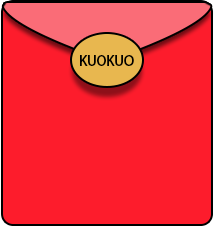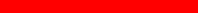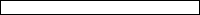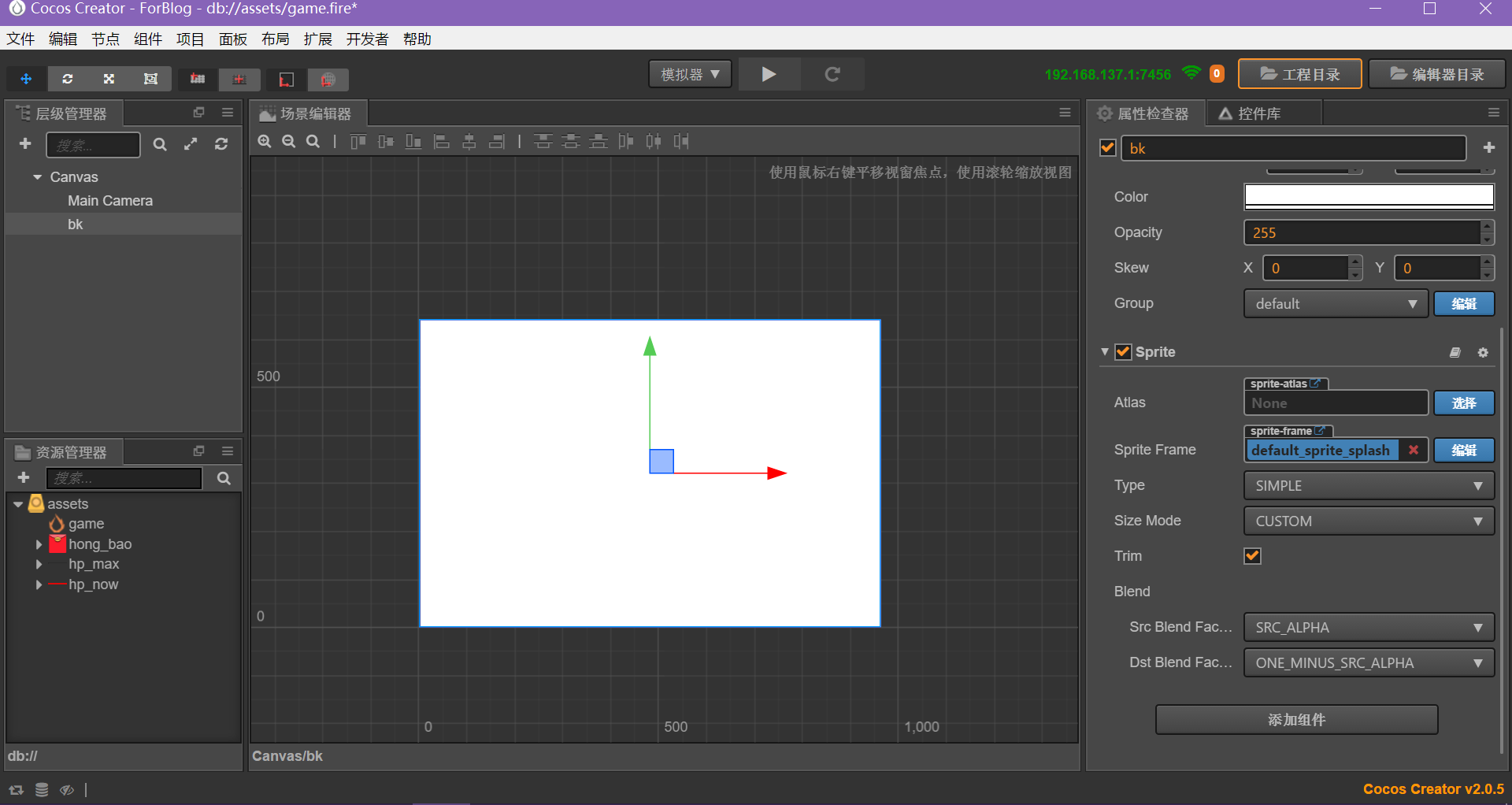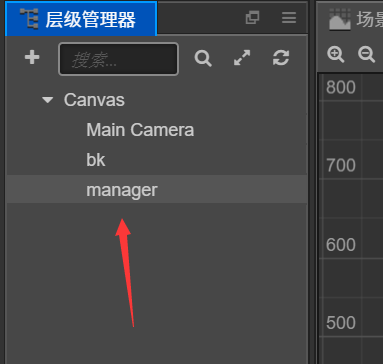然后，我们把红包放上去，y给-100：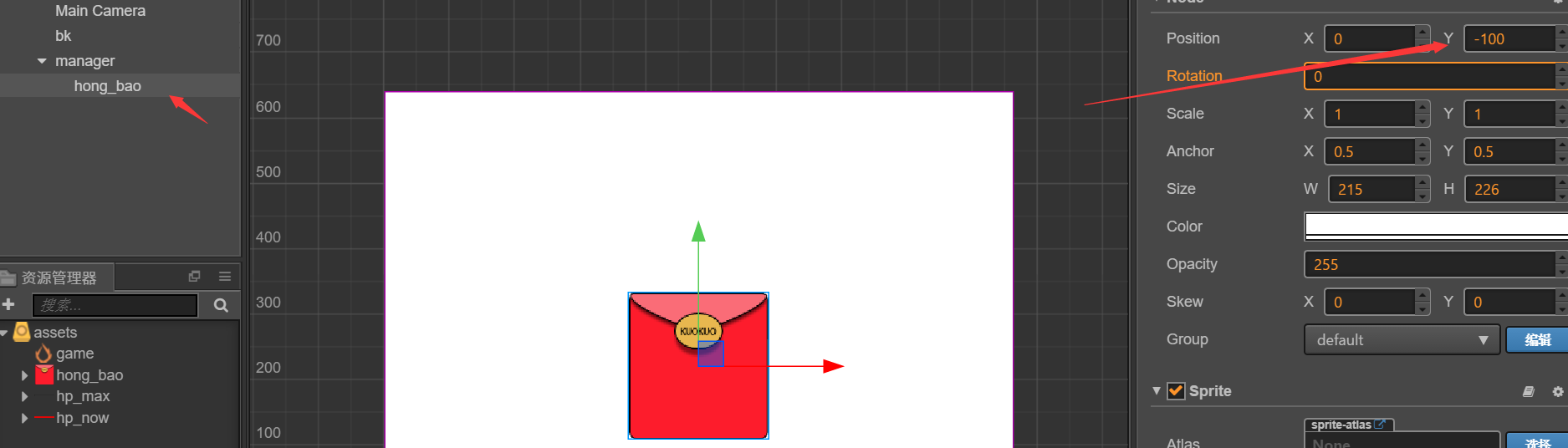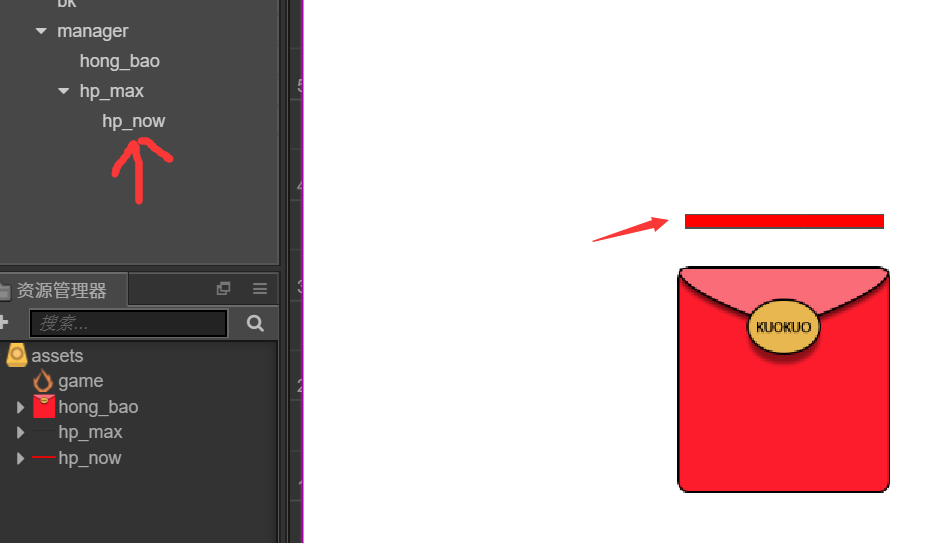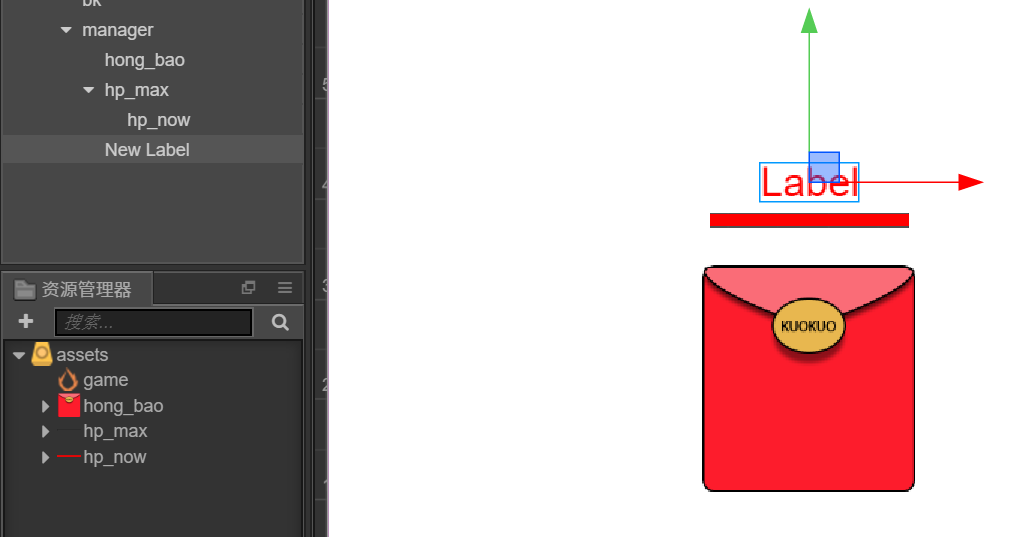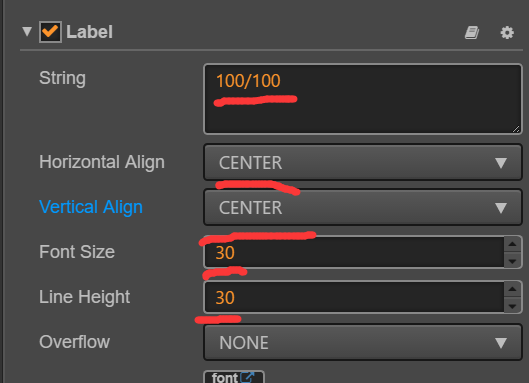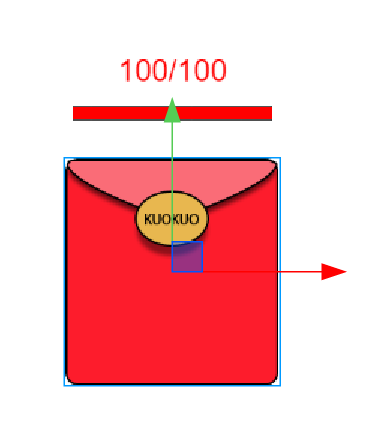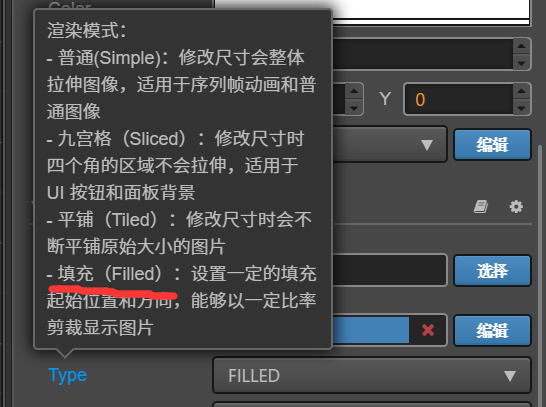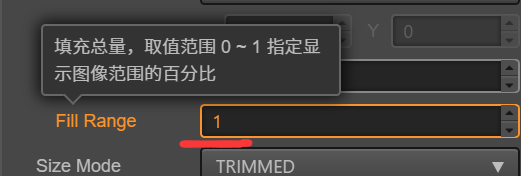0-1，可修改！

cc.Class({
extends: cc.Component,

properties: {

},

start () {
this.node.on('touchstart',function(){
// 抖动动作
// let 比 var 更严谨，用let
let act = cc.sequence(cc.moveBy(0.1,5,5),cc.moveBy(0.1,-5,-5));
this.node.runAction(act);
},this);
},
});


cc.Class({
extends: cc.Component,

properties: {
// 先声明label
hp_label : cc.Label,
},

// 最大血量
this.hp_max = 100;
// 目前血量
this.hp_now = 100;
// 每次攻击值
this.atk = 5;
// 初始化血量显示
this.hp_label.string = this.hp_now + '/' + this.hp_max;
},

start () {
// 触摸监听
this.node.on('touchstart',function(){
// 抖动效果
let act = cc.sequence(cc.moveBy(0.1,5,5),cc.moveBy(0.1,-5,-5));
this.node.runAction(act);
},this);
},
});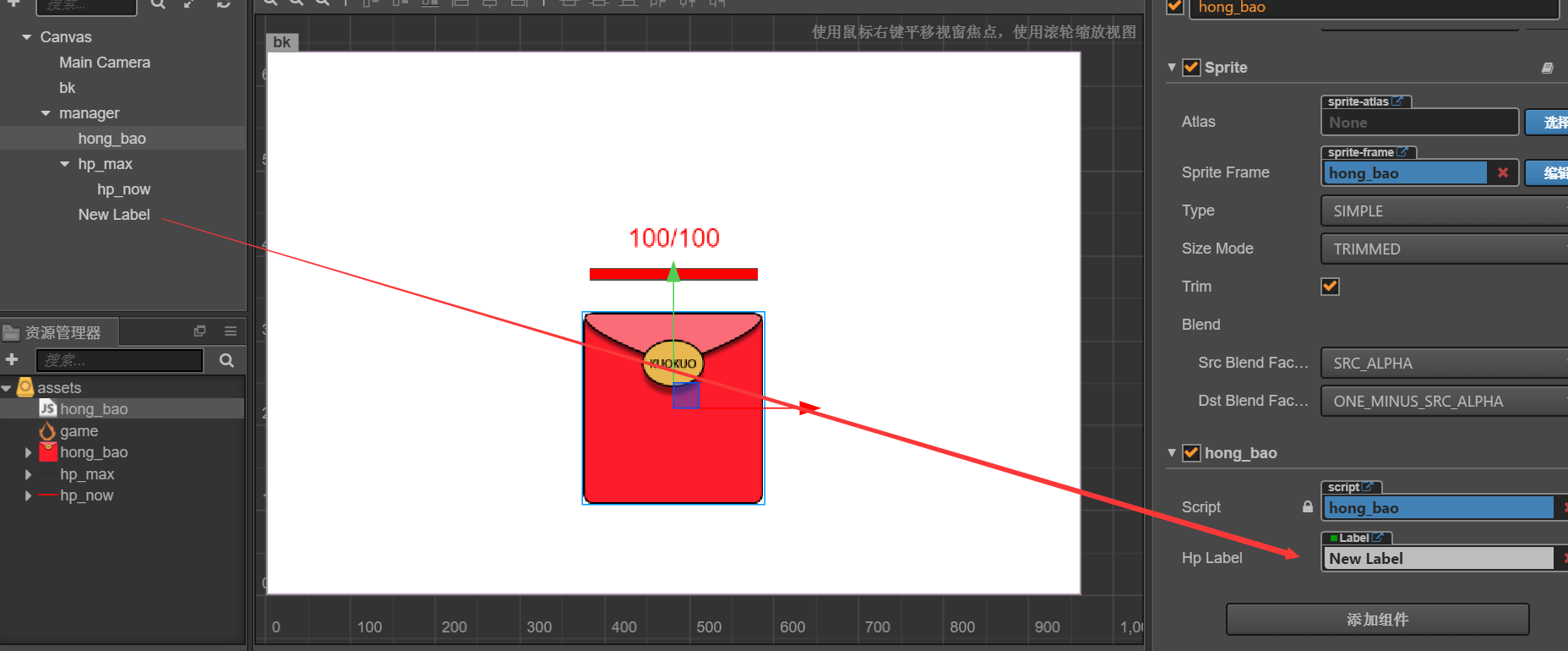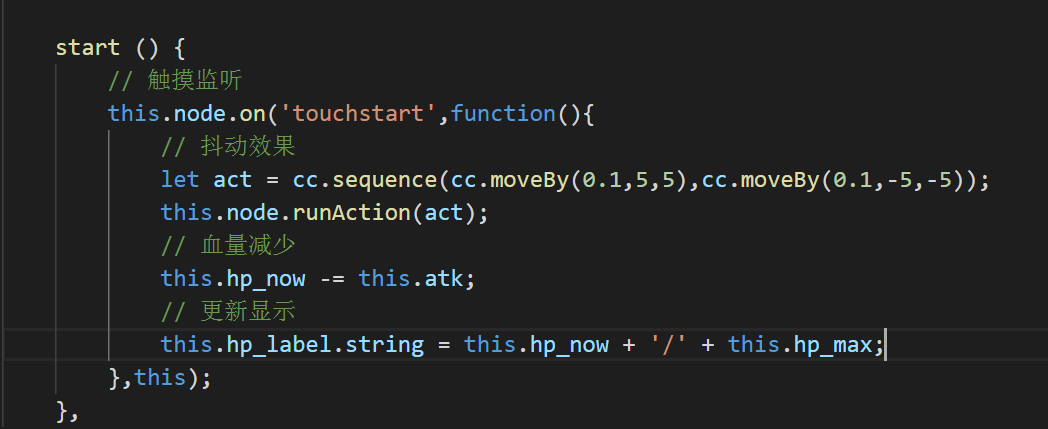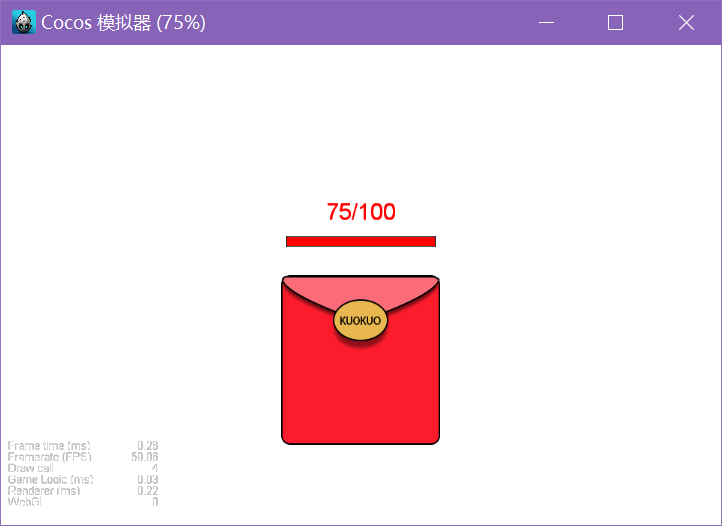（小伙伴们，谷歌浏览器应该是必备的哦）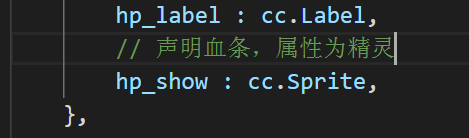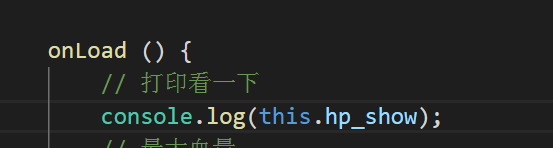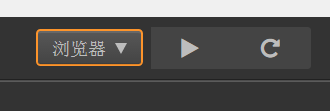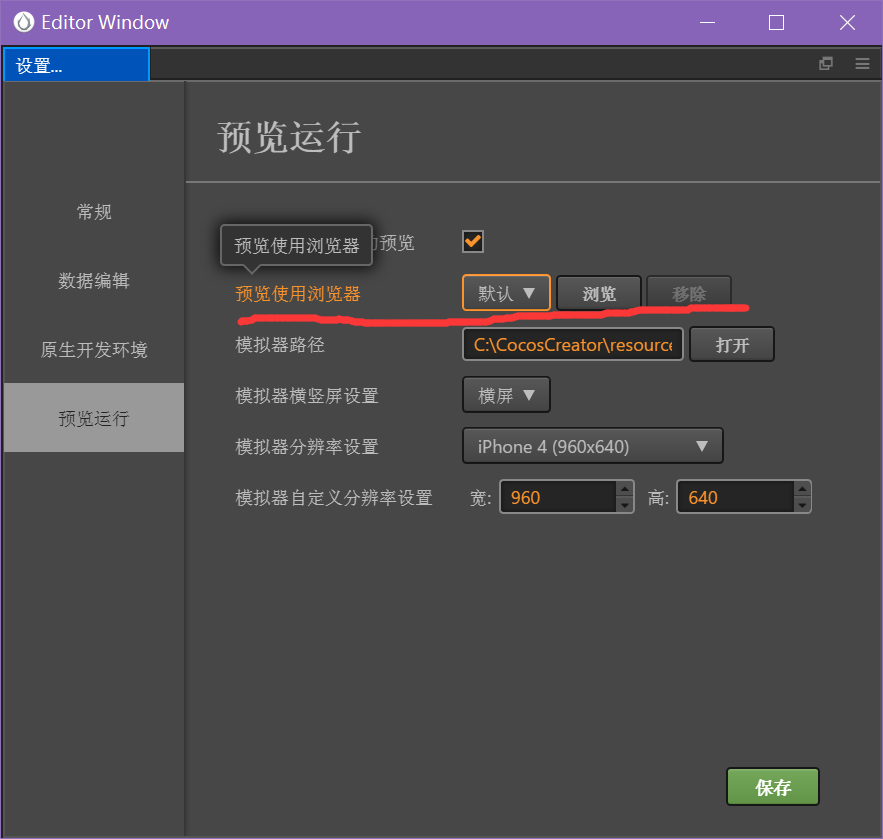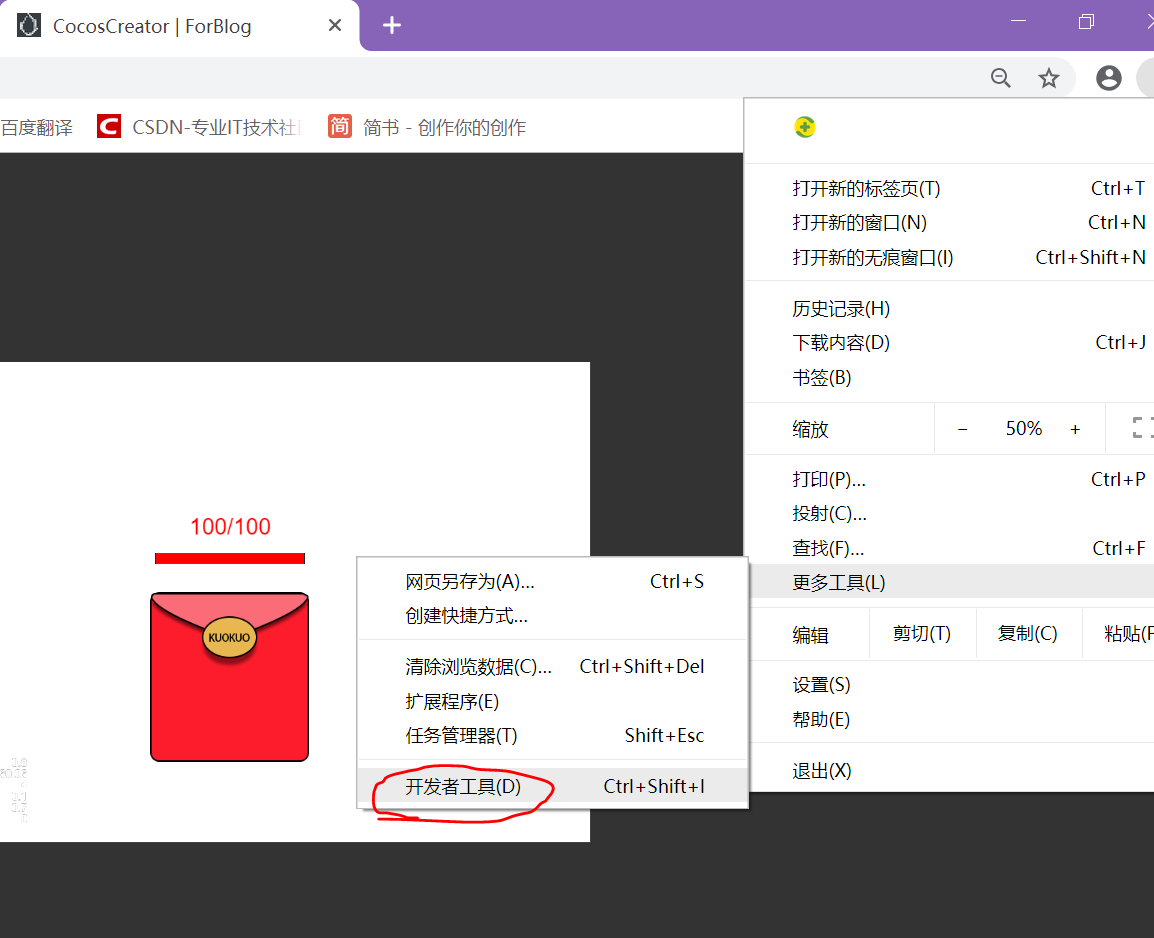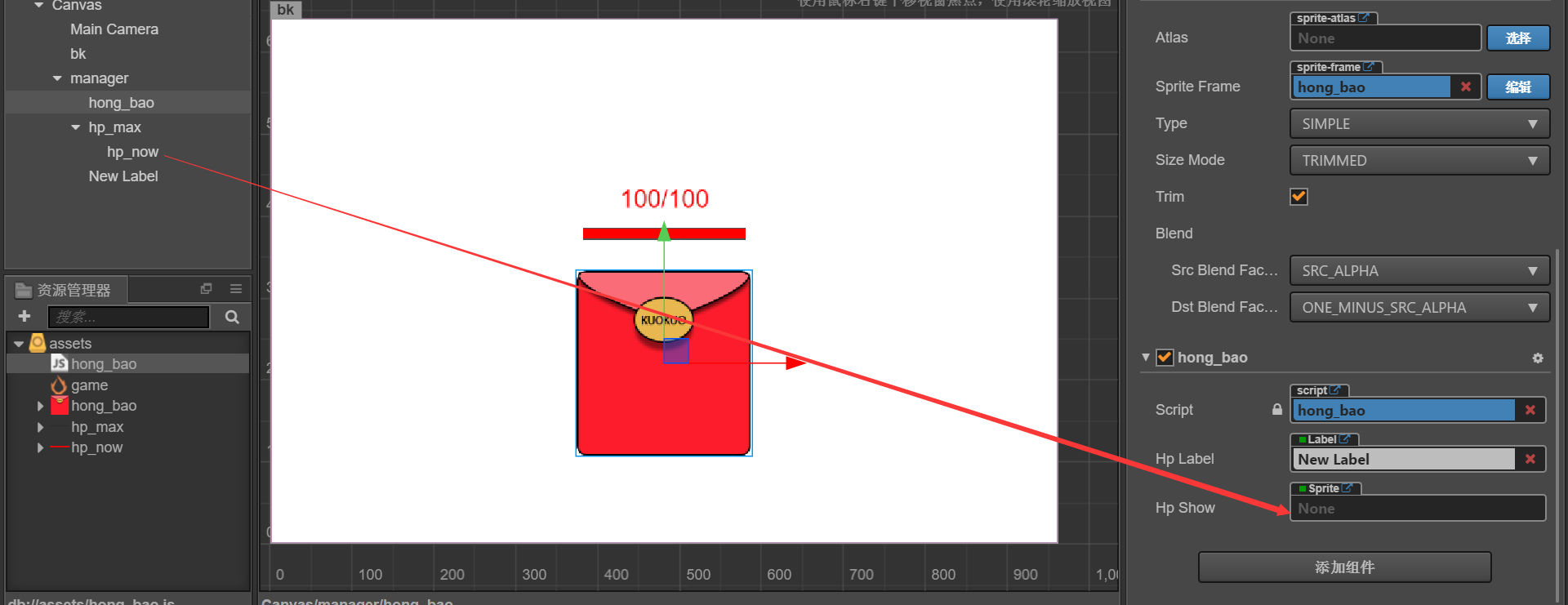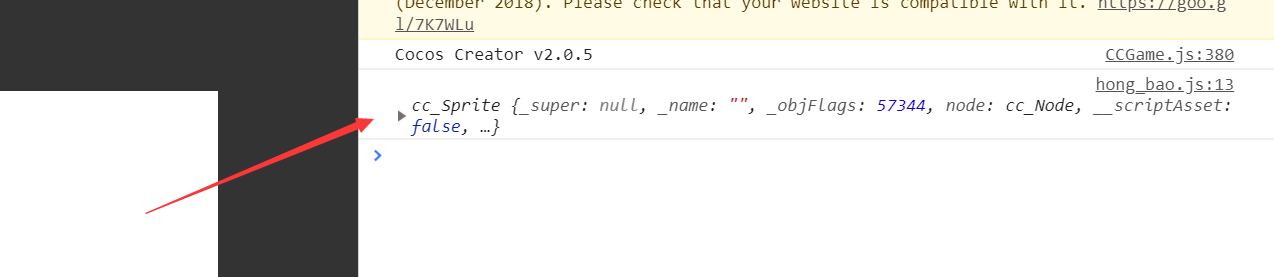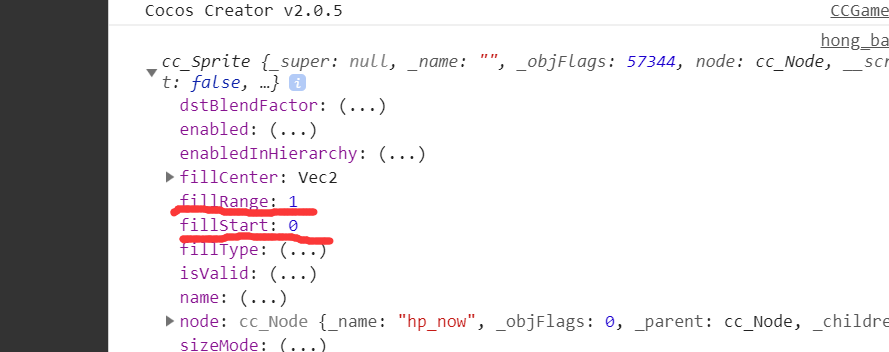cc.Class({
extends: cc.Component,

properties: {
// 先声明label
hp_label : cc.Label,
// 声明血条，属性为精灵
hp_show : cc.Sprite,
},

// 最大血量
this.hp_max = 100;
// 目前血量
this.hp_now = 100;
// 每次攻击值
this.atk = 5;
// 初始化血量显示
this.hp_label.string = this.hp_now + '/' + this.hp_max;
},

start () {
// 触摸监听
this.node.on('touchstart',function(){
// 抖动效果
let act = cc.sequence(cc.moveBy(0.1,5,5),cc.moveBy(0.1,-5,-5));
this.node.runAction(act);
// 血量减少
this.hp_now -= this.atk;
// 更新显示
this.hp_label.string = this.hp_now + '/' + this.hp_max;
// 求出血量占比
let hp_bili = this.hp_now / this.hp_max;
// 更改填充范围
this.hp_show.fillRange = hp_bili;
},this);
},
});


OK，让我们试试效果：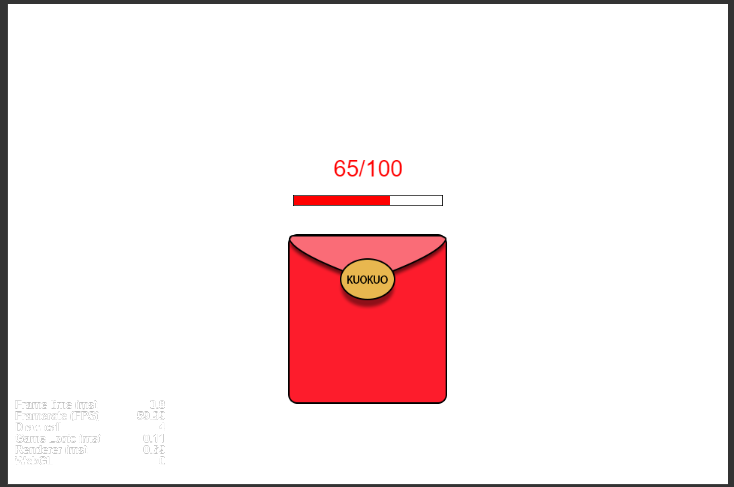706176551

O(∩_∩)O~~

©️2019 CSDN 皮肤主题: 书香水墨 设计师: CSDN官方博客